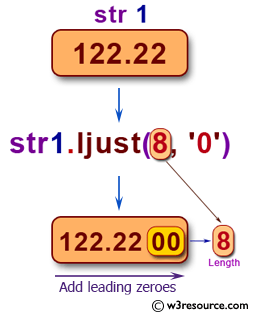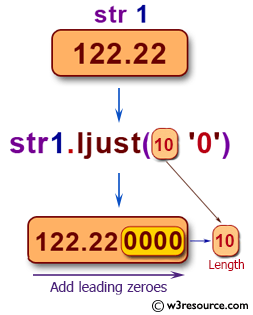## Python Basic: Exercise-129 with Solution

Pictorial Presentation:Sample Solution-1:

Python Code:

``````str1='122.22'
print("Original String: ",str1)
str1 = str1.ljust(8, '0')
print(str1)
str1 = str1.ljust(10, '0')
print(str1)
str1='122.22'
str1 = str1.rjust(8, '0')
print(str1)
str1 = str1.rjust(10, '0')
print(str1)
```
```

Sample Output:

```Original String:  122.22

122.2200
122.220000

00122.22
0000122.22
```

## Visualize Python code execution:

The following tool visualize what the computer is doing step-by-step as it executes the said program:

Sample Solution-2:

Python Code:

``````str1='122.22'
print("Original String: ",str1)
f_text = '{:<08}'
str1 = f_text.format(str1)
print(str1)
f_text = '{:<010}'
str1 = f_text.format(str1)
print(str1)
str1='122.22'
f_text = '{:>08}'
str1 = f_text.format(str1)
print(str1)
f_text = '{:>010}'
str1 = f_text.format(str1)
print(str1)
```
```

Sample Output:

```Original String:  122.22

122.2200
122.220000

00122.22
0000122.22
```

## Visualize Python code execution:

The following tool visualize what the computer is doing step-by-step as it executes the said program:

Python Code Editor:

Have another way to solve this solution? Contribute your code (and comments) through Disqus.

What is the difficulty level of this exercise?

Test your Python skills with w3resource's quiz

﻿

## Python: Tips of the Day

Try-catch-else construct:

```try:
foo()
except Exception:
print("Exception occured")
else:
print("Exception didnt occur")
finally:
print("Always gets here")
```